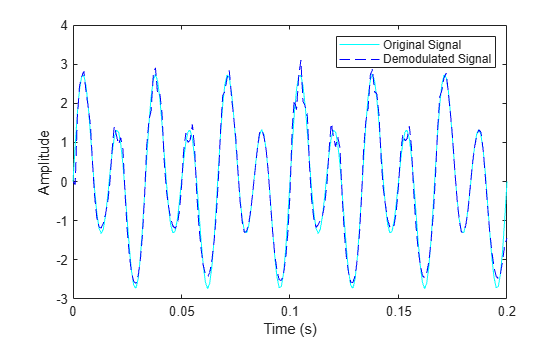fmdemod

Frequency demodulation

Description

example

z = fmdemod(y,Fc,Fs,freqdev) returns a demodulated signal z, given the input frequency modulated (FM) signal y, where the carrier signal has frequency Fc and sampling rate Fs. freqdev is the frequency deviation of the modulated signal.

Note

• The value of Fs must satisfy Fs ≥ 2Fc.

• The value of freqdev must satisfy freqdev < Fc.

z = fmdemod(y,Fc,Fs,freqdev,ini_phase) specifies the initial phase of the modulated signal in radians.

Examples

collapse all

Set the sampling frequency to 1kHz and carrier frequency to 200 Hz. Generate a time vector having a duration of 0.2 s.

fs = 1000;
fc = 200;
t = (0:1/fs:0.2)';

Create two-tone sinusoidal signal with frequencies 30 and 60 Hz.

x = sin(2*pi*30*t)+2*sin(2*pi*60*t);

Set the frequency deviation to 50 Hz.

fDev = 50;

Frequency modulate x.

y = fmmod(x,fc,fs,fDev);

Demodulate z.

z = fmdemod(y,fc,fs,fDev);

Plot the original and demodulated signals.

plot(t,x,'c',t,z,'b--');
xlabel('Time (s)')
ylabel('Amplitude')
legend('Original Signal','Demodulated Signal')The demodulated signal closely approximates the original.

Input Arguments

collapse all

Frequency modulated input signal, specified as a scalar, vector, matrix, or 3-D array. Each element of y must be real.

Data Types: double | single

Carrier frequency in hertz (Hz), specified as a positive real scalar.

Data Types: double

Sampling rate in hertz (Hz), specified as a positive scalar.

Data Types: double

Frequency deviation of the modulated signal in hertz (Hz), specified as a positive scalar.

Data Types: double

Initial phase of the modulated signal in radians, specified as a scalar.

Data Types: double

Output Arguments

collapse all

Frequency demodulated signal, returned as a scalar, vector, matrix, or 3-D array.